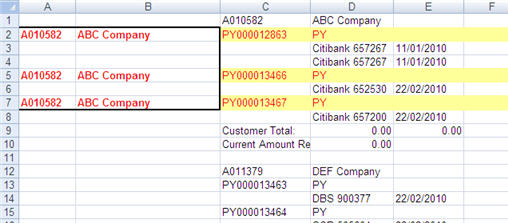Question:

The worksheet shows some payments under each customer (header). How can I apply the “sum if” formula to copy the customer code and customer name to every “PY” in Column D?You cannot apply SUMIF to copy the customer code and company name to every PY in column D. The formula you should use is IF.

First, you must put the customer code and company in the first row. The best way to do this is to use the formula method. For example, in A1 put the formula =C1 to display the customer code in A1.

Using the IF formula, we can set the condition to pick up the customer code or the company name is column D contains “PY”, i.e. in cell A1, enter the formula

=IF(D2=”PY”,A1,….)

That is the first part.

Now the IF formula has a part to present a value when column D does not contain the text “PY”. Specifcally, we are interested in is to return the company name if it ever changes and discards all other values. To do this, we have to find the pattern that we can use to identify that the row contains company name. We can check whether column E is empty. We can use another IF formula to do this. Since we have dealt with “PY” in column D, it will not pick up “PY” again. In this case, it will pick up the customer code when it detect the column E is empty. So we have to add another IF formula into the first IF formula. Now the formula becomes

=IF(D2=”PY”,A1,IF(E2=””,C2,….

If it does not find a blank, return the value that is above the formula, i.e. A1. So the the formula in A2 finally becomes

=IF(D2=”PY”,A1,IF(E2=””,C2,A1))